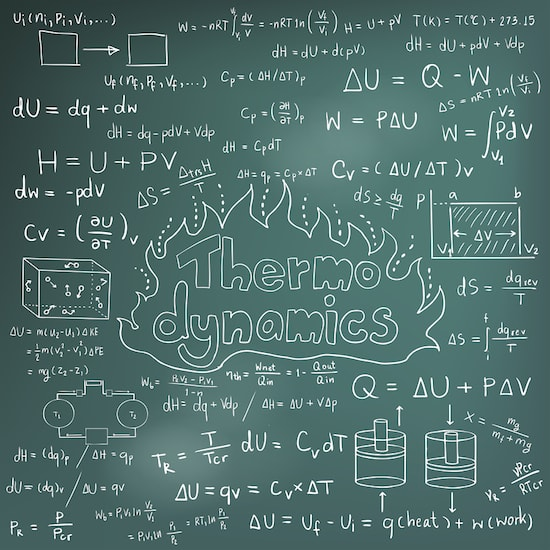As a JC level student, you probably need no introduction to thermodynamics. In case you need a reminder, thermodynamics is the study of heat and temperature in relation to work and energy. Thermodynamics is concerned only with changes and observations on a macroscopic (large-scale) level.
There are four laws of thermodynamics that every JC level student must understand.
Zeroth Law of Thermodynamics
This law states that if two separate systems are in thermal equilibrium with a third system, they are also in thermal equilibrium with each other. It’s like saying if A = C and B = C, then A = B.
Let’s take two beakers of warmed water as an example. Take a thermometer and put it in the first beaker. Let’s say the thermometer reads 80 degrees Celsius and goes no higher. The thermometer is in thermal equilibrium with the first beaker. Now put the thermometer in the second beaker. Let’s say it continues to read 80 degrees Celsius. The thermometer is in thermal equilibrium with the second beaker.
Since both beakers are in thermal equilibrium with the thermometer, we can say that the two beakers are in thermal equilibrium with each other.
First Law of Thermodynamics
This law states that every thermodynamic system possesses an internal energy and the change in the internal energy of the system is equal to the heat added to the system minus the work done by the system. This makes it an extension of the law of conservation of energy.
What this law really means is that energy cannot be created or destroyed, it can only be changed from one form to another (e.g., heat energy to mechanical energy). The total amount of energy in the universe remains the same; it only changes from one form to another.
Let’s take a car engine as an example. When the engine is running, the fuel is burned to change the chemical energy stored in it to heat energy, a large portion of which is changed into mechanical energy. Some heat dissipates into the surrounding and some changes into sound.
When all the fuel is burned up, the total amount of heat energy that was released into the engine is equal to the sum of the mechanical energy, heat energy and sound energy that was produced. No new energy was created and no existing energy was destroyed in the process.
Second Law of Thermodynamics
This law states that entropy always increases with time in a thermodynamic system. Entropy is a measure of a system’s thermal energy that is lost to the surroundings and is, thus, unavailable for useful work. In simple words, heat energy flows from an area of high temperature to an area of low temperature until they reach a state of thermal equilibrium.
Let’s take a cup of boiled water placed on a table in a room as an example. The water slowly loses heat to the surrounding. Give it sufficient time and the water will have same temperature as the air in the room, i.e., it will be in a state of thermal equilibrium with the air in the room.
Third Law of Thermodynamics
This law states that the entropy of a system approaches a value that is equal to zero as the temperature approaches to absolute zero. In simpler words, the lower the temperature of a thermal system, the less the entropy. For the entropy to be equal to or very near to zero, the thermal body must be a perfect crystal. Absolute zero is minus 273.15 C (minus 459.67 F).
Thermodynamics can be tough nut to crack but with help from physics tuition, it will be easier to understand. If you are thinking of taking physics tuition, make sure to take it from a renowned centre in Singapore.